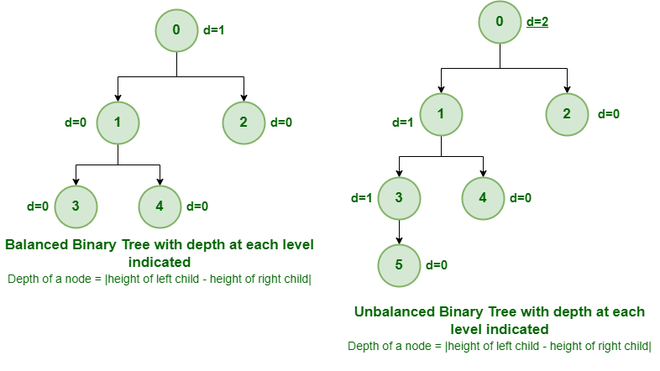# Binary Tree | Set 3 (Types of Binary Tree)

• Difficulty Level : Easy
• Last Updated : 27 Jun, 2022

We have discussed Introduction to Binary Tree in set 1 and Properties of Binary Tree in Set 2. In this post, common types of Binary Trees are discussed.

The following are common types of Binary Trees.

Full Binary Tree:

A Binary Tree is a full binary tree if every node has 0 or 2 children. The following are the examples of a full binary tree. We can also say a full binary tree is a binary tree in which all nodes except leaf nodes have two children.

A full Binary tree is a special type of binary tree in which every parent node/internal node has either two or no children. It is also known as a proper binary tree.

```               18
/       \
15         30
/  \        /  \
40    50    100   40

18
/    \
15     20
/  \
40    50
/   \
30   50

18
/     \
40       30
/  \
100   40```

Complete Binary Tree:-

A Binary Tree is a Complete Binary Tree if all the levels are completely filled except possibly the last level and the last level has all keys as left as possible.

A complete binary tree is just like a full binary tree, but with two major differences:

• Every level must be completely filled
• All the leaf elements must lean towards the left.
• The last leaf element might not have a right sibling i.e. a complete binary tree doesn’t have to be a full binary tree.

The following are examples of Complete Binary Trees.

```               18
/       \
15         30
/  \        /  \
40    50    100   40

18
/       \
15         30
/  \        /  \
40    50    100   40
/  \   /
8   7  9 ```

Practical example of Complete Binary Tree is Binary Heap

Perfect Binary Tree:-

A Binary tree is a Perfect Binary Tree in which all the internal nodes have two children and all leaf nodes are at the same level.
The following are the examples of Perfect Binary Trees.

A perfect binary tree is a type of binary tree in which every internal node has exactly two child nodes and all the leaf nodes are at the same level.

```               18
/       \
15         30
/  \        /  \
40    50    100   40

18
/       \
15         30  ```

In a Perfect Binary Tree, the number of leaf nodes is the number of internal nodes plus 1

L = I + 1 Where L = Number of leaf nodes, I = Number of internal nodes.

A Perfect Binary Tree of height h (where the height of the binary tree is the number of edges in the longest path from the root node to any leaf node in the tree, height of root node is 0) has 2h+1 – 1 node.

An example of a Perfect binary tree is ancestors in the family. Keep a person at root, parents as children, parents of parents as their children.

Balanced Binary Tree:-
A binary tree is balanced if the height of the tree is O(Log n) where n is the number of nodes. For Example, the AVL tree maintains O(Log n) height by making sure that the difference between the heights of the left and right subtrees is at most 1. Red-Black trees maintain O(Log n) height by making sure that the number of Black nodes on every root to leaf paths is the same and there are no adjacent red nodes. Balanced Binary Search trees are performance-wise good as they provide O(log n) time for search, insert and delete.Example of Balanced and Unbalanced Binary Tree

It is a type of binary tree in which the difference between the height of the left and the right subtree for each node is either 0 or 1. In the figure above, the root node having a value 0 is unbalanced with a depth of 2 units.

A degenerate (or pathological) tree:-

A Tree where every internal node has one child. Such trees are performance-wise same as linked list.

A degenerate or pathological tree is the tree having a single child either left or right.

```      10
/
20
\
30
\
40     ```

Skewed Binary Tree:-

A skewed binary tree is a pathological/degenerate tree in which the tree is either dominated by the left nodes or the right nodes. Thus, there are two types of skewed binary tree: left-skewed binary tree and right-skewed binary tree.

```      10                                           10
/                                             \
20                                               20
/                                                 \
30                                                   30
/                                                     \
40                                                      40
Left-Skewed Binary Tree                               Right-Skewed Binary Tree```
My Personal Notes arrow_drop_up